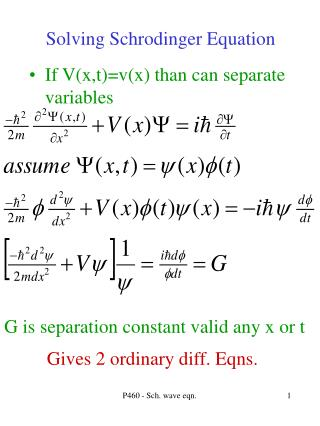DownloadDownload PresentationSolving Schrodinger Equation

# Solving Schrodinger Equation

Télécharger la présentation## Solving Schrodinger Equation

- - - - - - - - - - - - - - - - - - - - - - - - - - - E N D - - - - - - - - - - - - - - - - - - - - - - - - - - -
##### Presentation Transcript

1. Solving Schrodinger Equation If V(x,t)=v(x) than can separate variables G is separation constant valid any x or t Gives 2 ordinary diff. Eqns. P460 - Sch. wave eqn. 1

2. Solutions to Schrod Eqn Gives energy eigenvalues and eigenfunctions (wave functions). These are quantum states. Linear combinations of eigenfunctions are also solutions. For discrete solutions If H Hermitian P460 - Sch. wave eqn. 2

3. G=E if 2 energy states, interference/oscillation 1D time independent Scrod. Eqn. Solve: know U(x) and boundary conditions want mathematically well-behaved. Do not want: No discontinuities. Usually except if V=0 or y =0 in certain regions P460 - Sch. wave eqn. 3

4. Linear Operators Operator converts one function into another an operator is linear if (to see, substitute in a function) linear suppositions of eigenfunctions also solution if operator is linear……use “Linear algebra” concepts. Often use linear algebra to solve non-linear functions…. P460 - Sch. wave eqn. 4

5. Solutions to Schrod Eqn Depending on conditions, can have either discrete or continuous solutions or a combination where Cn and C(E) are determined by taking the dot product of an arbitrary function y with the eigenfunctions u. Any function in the space can be made from linear combinations P460 - Sch. wave eqn. 5

6. Solutions to Schrod Eqn Linear combinations of eigenfunctions are also solutions. Assume two energies assume know wave function at t=0 at later times the state can oscillate between the two states - probability to be at any x has a time dependence P460 - Sch. wave eqn. 6

7. Example 3-1 Boundary conditions (including the functions being mathematically well behaved) can cause only certain, discrete eigenfunctions solve eigenvalue equation impose the periodic condition to find the allowed eigenvalues P460 - Sch. wave eqn. 7

8. Square Well Potential Start with the simplest potential Boundary condition is that y is continuous:give: V 0 -a/2 a/2 P460 - Sch. wave eqn. 8

9. Infinite Square Well Potential Solve S.E. where V=0 Boundary condition quanitizes k/E, 2 classes Odd y=Asin(knx) kn=np/a n=2,4,6... y(x)=-y(-x) Even y=Bcos(knx) kn=np/a n=1,3,5... y(x)=y(-x) P460 - Sch. wave eqn. 9

10. Parity Parity operator P x  -x (mirror) determine eigenvalues even and odd functions are eigenfunctions of P any function can be split into even and odd P460 - Sch. wave eqn. 10

11. Parity If V(x) is an even function then H is also even then H and P commute and parity is a constant. If the initial state is even it stays even, odd stays odd. Semi-prove: time development of a wavefunction is given by do the same for Py when [H,P]=0 and so a state of definite parity (+,-) doesn’t change parity over time; parity is conserved (strong and EM forces conserve, weak force does not) P460 - Sch. wave eqn. 11

12. Infinite Square Well Potential Need to normalize the wavefunction. Look up in integral tables What is the minimum energy of an electron confined to a nucleus? Let a = 10-14m = 10 F P460 - Sch. wave eqn. 12

13. Infinite Square Well Density of States The density of states is an important item in determining the probability that an interaction or decay will occur it is defined as for the infinite well For electron with a = 1mm, what is the number of states within 0.0001 eV about 0.01 eV? P460 - Sch. wave eqn. 13

14. Example 3-5 Particle in box with width a and a wavefunction of Find the probability that a measurement of the energy gives the eigenvalue En With only n=odd only from the symmetry The probability to be in state n is then P460 - Sch. wave eqn. 14

15. Free particle wavefunction If V=0 everywhere then solutions are but the exponentials are also eigenfunctions of the momentum operator can use to describe left and right traveling waves book describes different normalization factors P460 - Sch. wave eqn. 15